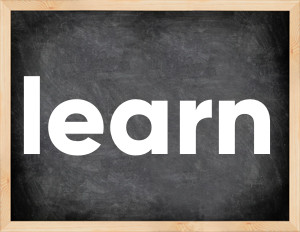# Learn past tenseThe English verb 'learn' is pronounced as [lɜ:n].
Related to: irregular verbs, regular verbs.
3 forms of verb learn: Infinitive (learn), Past Simple - (learnt), Past Participle - (learnt).

## Here are the past tense forms of the verb learn

👉 Forms of verb learn in future and past simple and past participle.
❓ What is the past tense of learn.

## Learn: Past, Present, and Participle Forms

Base Form Past Simple Past Participle
learn [lɜ:n]

learnt [lɜ:nt]

learned [lɜ:nd]

learnt [lɜ:nt]

learned [lɜ:nd]

## What are the 2nd and 3rd forms of the verb learn?

🎓 What are the past simple, future simple, present perfect, past perfect, and future perfect forms of the base form (infinitive) 'learn'?

### Learn the three forms of the English verb 'learn'

• the first form (V1) is 'learn' used in present simple and future simple tenses.
• the second form (V2) is 'learnt', 'learned' used in past simple tense.
• the third form (V3) is 'learnt', 'learned' used in present perfect and past perfect tenses.

## What are the past tense and past participle of learn?

The past tense and past participle of learn are: learn in past simple is learnt, learned, and past participle is learnt. learned.

### What is the past tense of learn?

The past tense of the verb "learn" is "learnt", or "learned", and the past participle is "learnt" or "learned".

### Verb Tenses

Past simple — learn in past simple learnt, learned (V2).
Future simple — learn in future simple is learn (will + V1).
Present Perfect — learn in present perfect tense is learnt, learned (have/has + V3).
Past Perfect — learn in past perfect tense is learnt, learned (had + V3).

### learn regular or irregular verb?

👉 Is 'learn' a regular or irregular verb? The verb 'learn' is regular or irregular verb.

## Examples of Verb learn in Sentences

•   You should live and learn! (Present Simple)
•   He is learning to drive (Present Continuous)
•   She had learned Sanskrit (Past Perfect)
•   Can't learn these new words (Present Simple)
•   Have you already learned these words? (Present Perfect)
•   And we all will learn something new (Future Simple)
•   Think of what we can learn here (Present Simple)
•   Today we have learned more than usually (Present Perfect)
•   I have learned from my own mistakes (Present Perfect)
•   You will never learn to cook if avoid a kitchen (Future Simple)

Along with learn, words are popular try and go.

Verbs by letter: , , , , , , , , , , , , , , , , , , , , , , , , .